• 时间序列分析-------乘法季节模型 实验 名称 乘法季节模型 实验 内容 乘法季节模型 实验 目的 2、熟练建立乘法季节模型 乘法季节...
时间序列分析-------乘法季节模型

实验

名称

乘法季节模型

实验

内容

乘法季节模型

实验

目的

2、熟练建立乘法季节模型

推荐阅读

使用Python完成时间序列分析基础
SPSS建立时间序列乘法季节模型实战案例
SPSS建立时间序列加法季节模型实战案例
Python建立时间序列ARIMA模型实战案例
乘法季节模型结构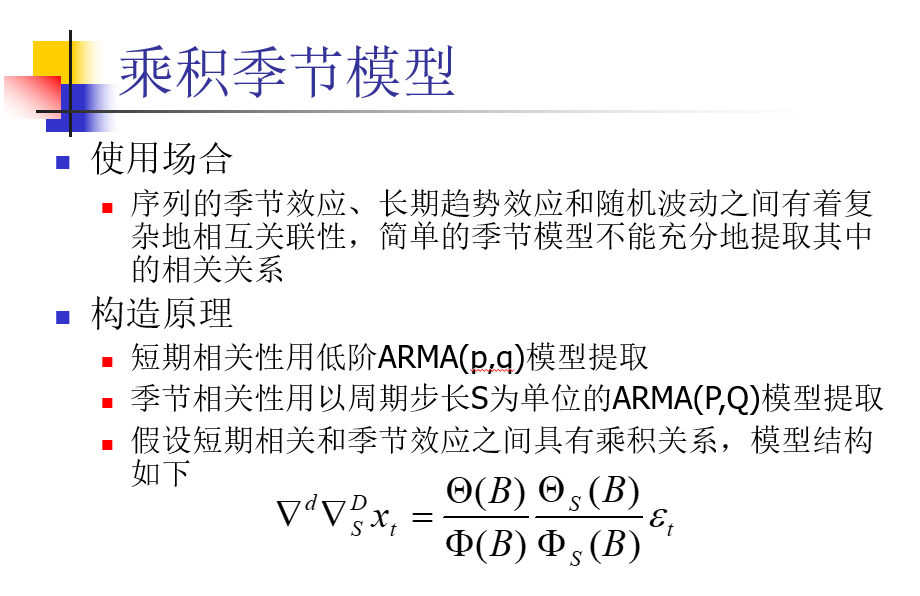乘法季节模型建立

绘制时序图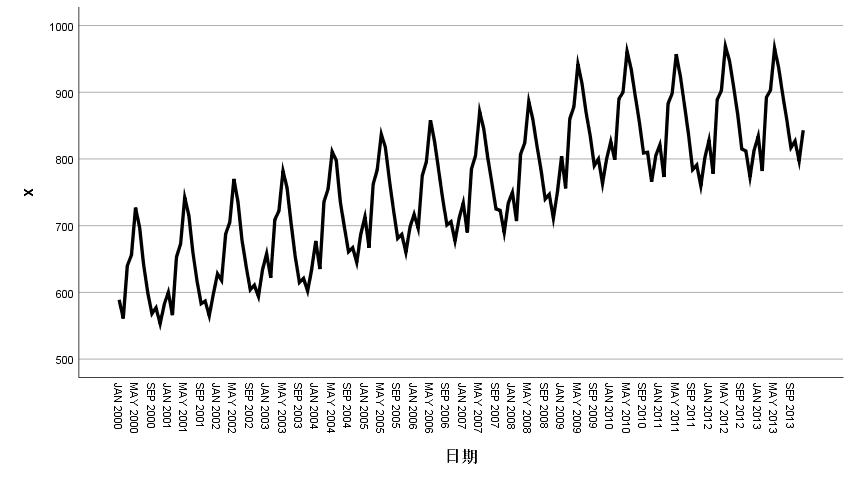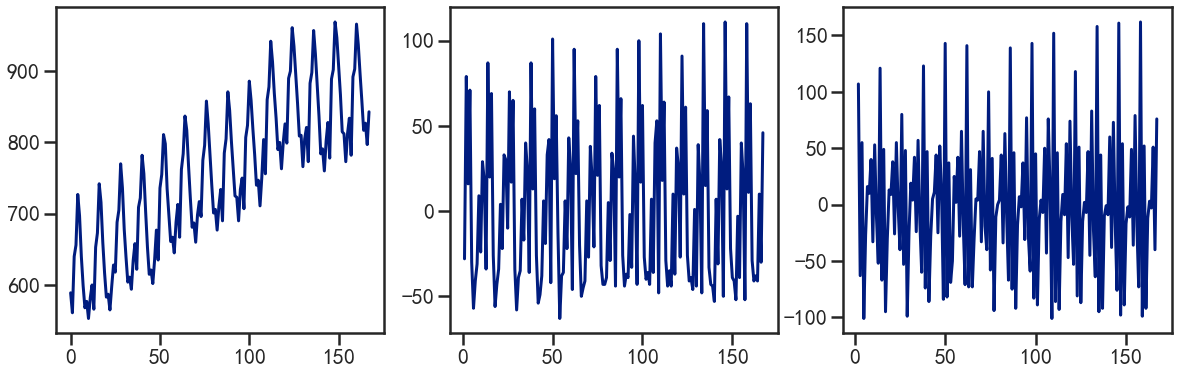时序图显示该序列具有长期增长性趋势和以年为周期的季节效应

差分平稳化

对原序列做1阶12步差分，希望提取原序列的趋势效应和季节效应，差分后的时序图如下所示：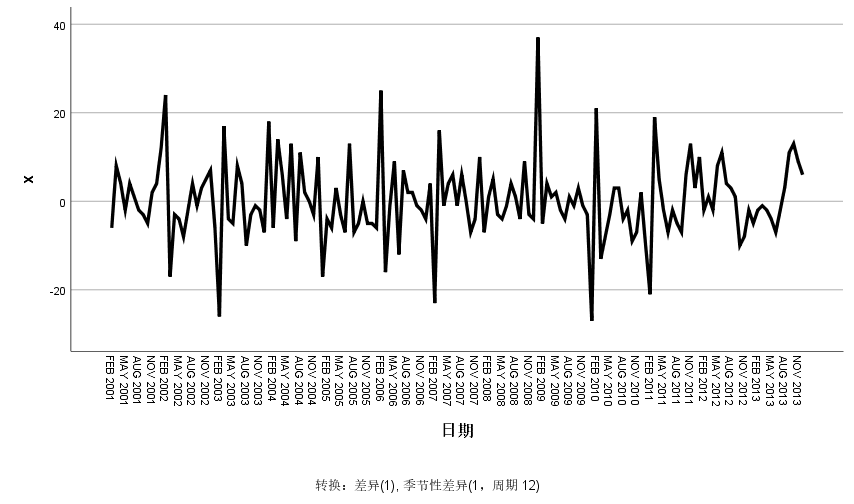易得模型平稳

白噪声检验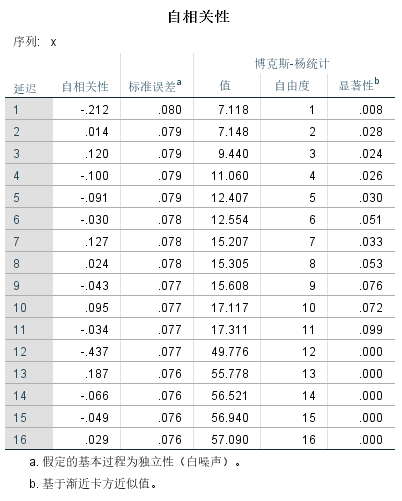P<α，故拒绝原假设，即差分后的序列属于非白噪声序列

综上：差分后的序列是平稳非白噪声序列，需要对差分后的序列进一步拟合ARMA模型

时序图定阶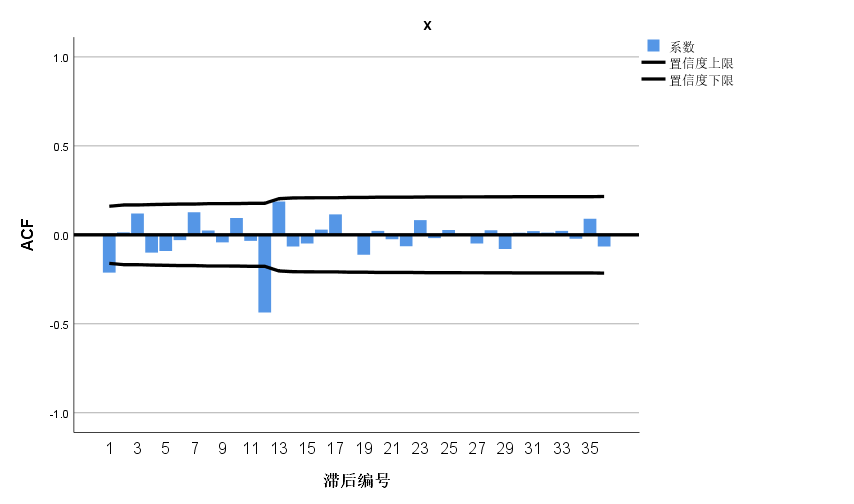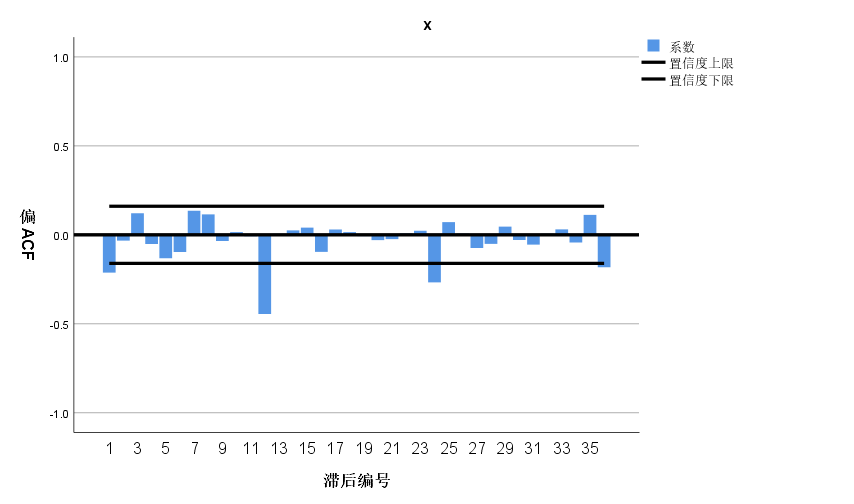自相关图显示延迟12阶自相关系数大于两倍标准差，这说明差分后序列中仍然蕴含非常显著的季节效应，延迟1阶的自相关系数也大于2倍标准差，这说明差分后序列还具有短期相关性。

短期相关特征：自相关图和偏自相关图显示12阶以内的自相关系数，ARMA(1,1),ARMA(1,0),ARMA(0,1)提取差分后序列的短期自相关信息
季节自相关特征：季节自相关特征时自相关系数截尾，偏自相关系数拖尾，这里可以永12步为周期的ARMA(0,1)12模型来提取差分后序列的季节自相关信息。
参数估计和模型检验

综合前面的差分信息，我们要建立ARIMA(1,1,1)*(0,1,1)12、ARIMA(1,1,0)*(0,1,1)12、ARIMA(0,1,1)*(0,1,1)12模型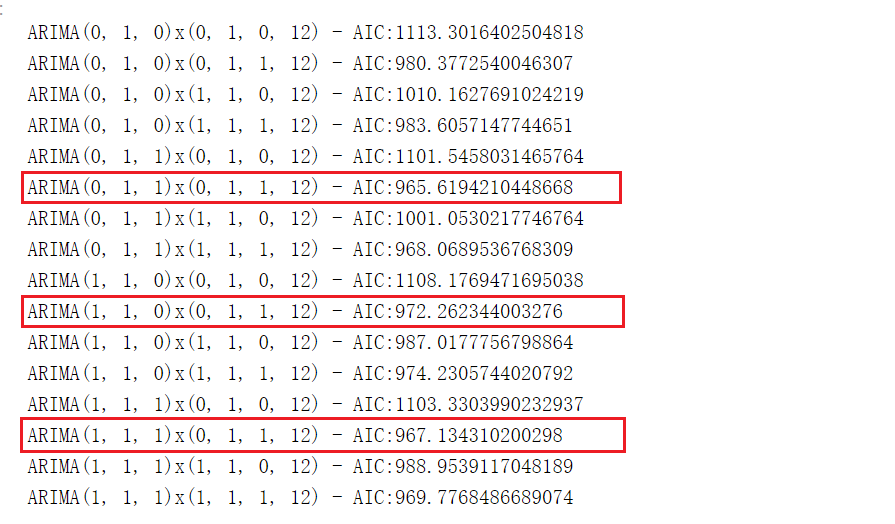ARIMA(1,1,1)*(0,1,1)12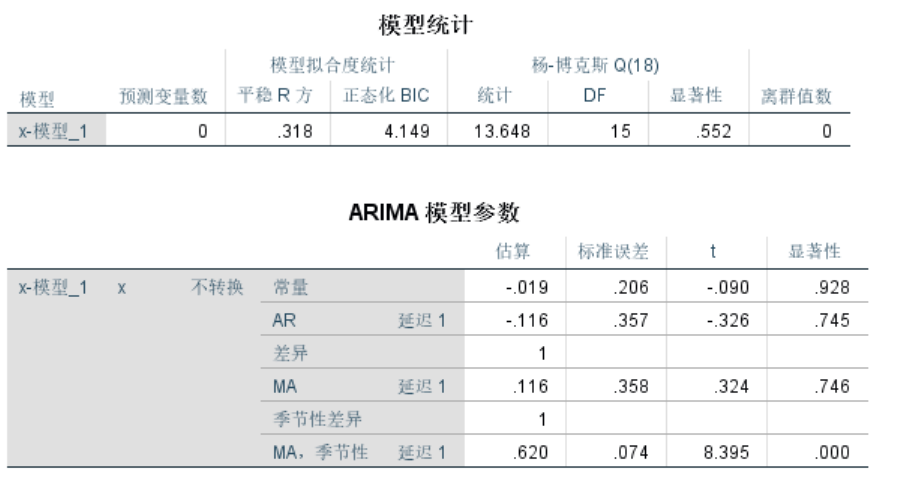P<α，参数的显著性不通过检验

ARIMA(0,1,1)*(0,1,1)12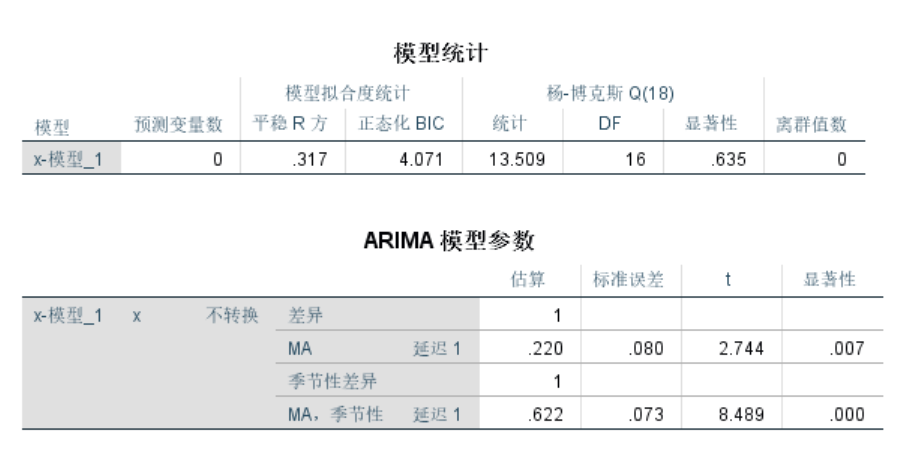模型的显著性检验：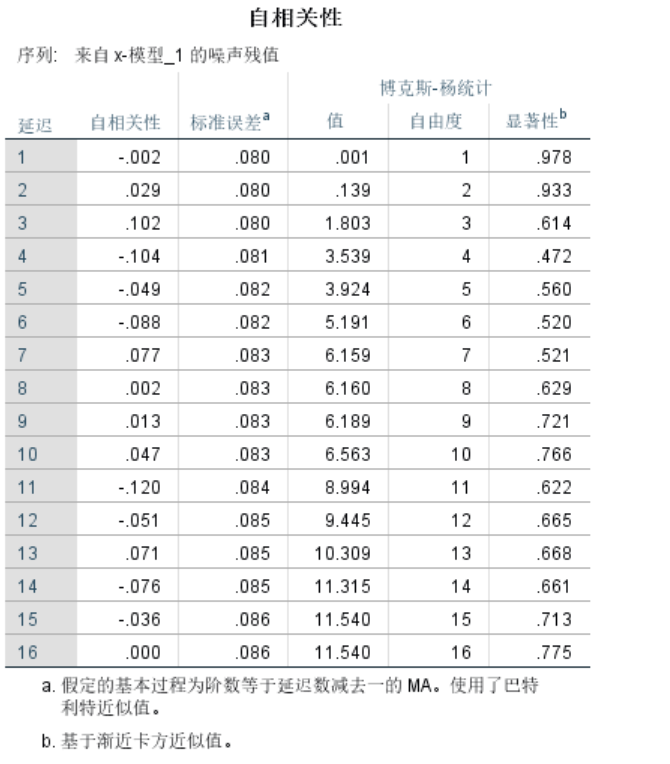建立模型：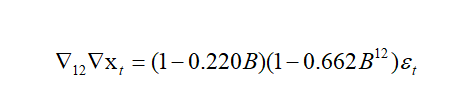ARIMA(1,1,0)*(0,1,1)12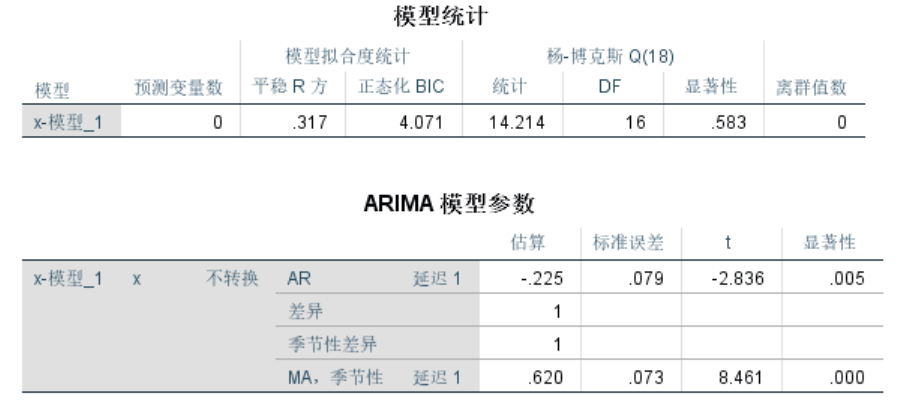参数的显著性检验通过

模型的显著性检验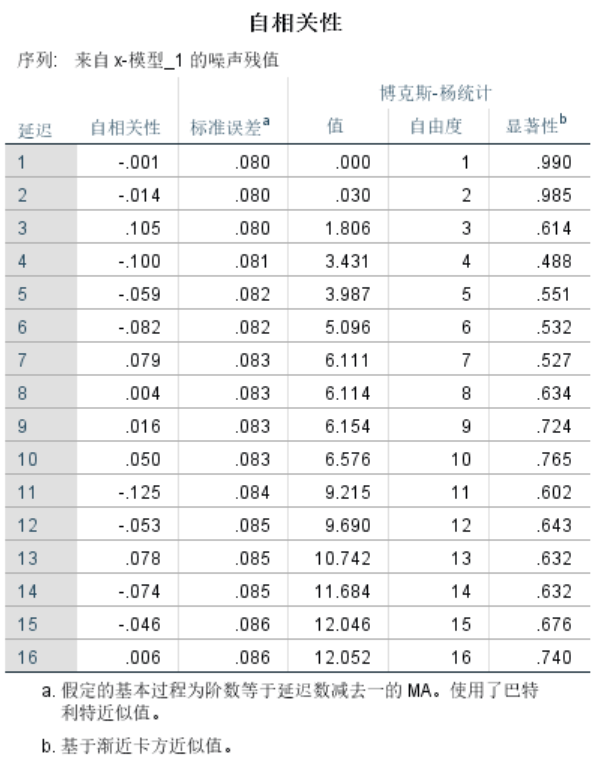建立模型：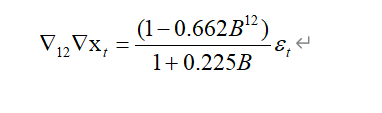模型预测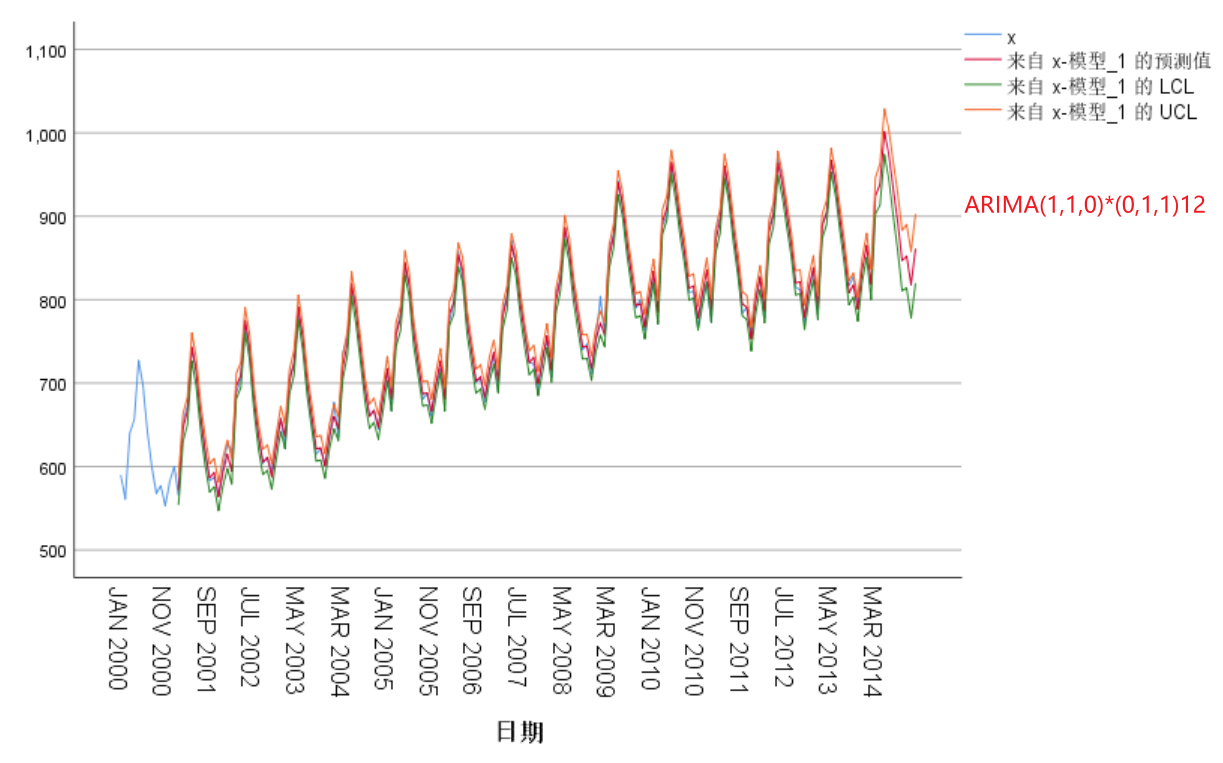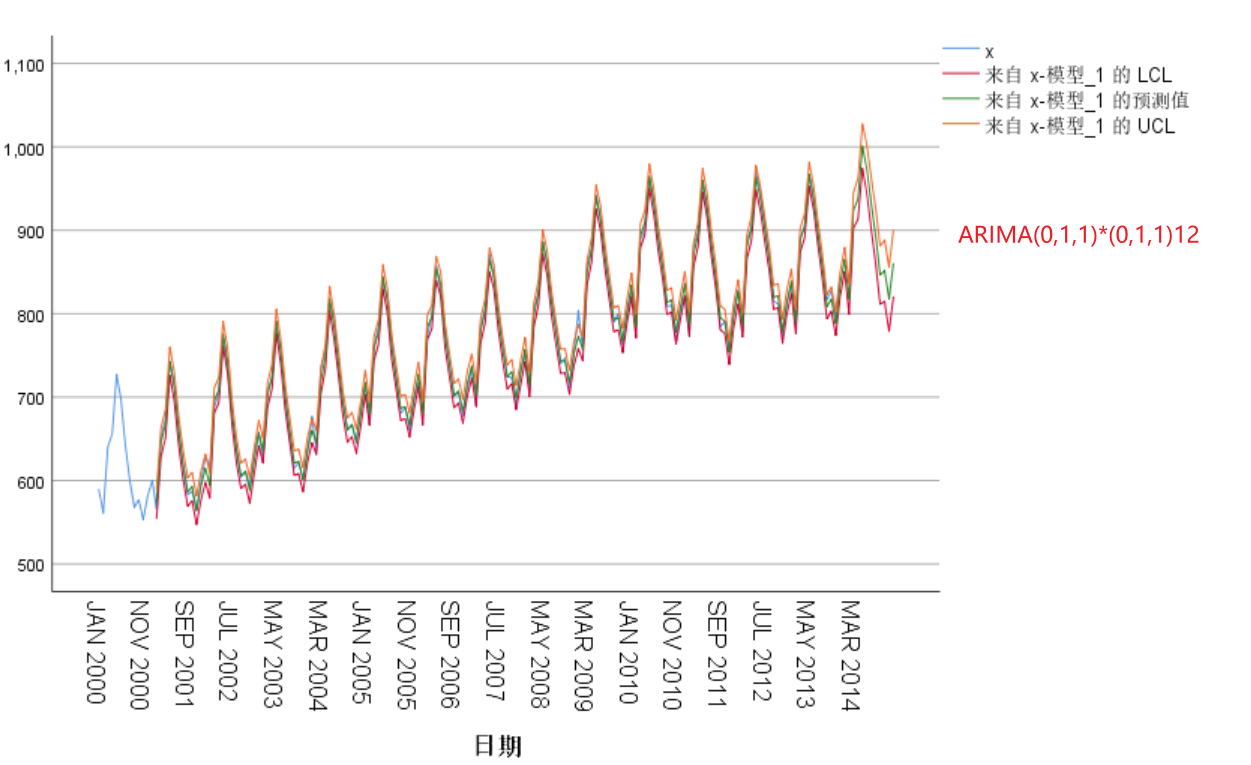> 到这里就结束了，如果对你有帮助你，欢迎点赞关注，你的点赞对我很重要


展开全文数据分析
• 时间序列分解是时间序列分析的一种方法，思想是将数据分解为不同的因素，以达到解释数据、建立数学模型、数据预测的目的，时间序列分解的方法有很多，较常用的模型有加法模型和乘法模型，这里介绍乘法模型乘法模型...
目录

方法介绍

数学公式

因素求解

案例

计算步骤

应用-预测

应用-异常检测

方法介绍

时间序列分解是时间序列分析的一种方法，思想是将数据分解为不同的因素，以达到解释数据、建立数学模型、数据预测的目的，时间序列分解的方法有很多，较常用的模型有加法模型和乘法模型，这里介绍乘法模型。乘法模型将时间序列值分解为长期趋势因素（）、季节变动因素（）、循环变动因素（）、不规则变动因素（，也可理解为随机因素）

数学公式

其中：

：第t期的值

：第t期的长期趋势值

：第t期的季节变动因素

：第t期的循环变动因素

：第t期的不规则变动因素（随机因素），服从正态分布

因素求解

1、移动平均提取趋势和循环变动：

当n为奇数时：，k是季节长度，例如每周7天，每年12个月等，下同

当n为偶数时：

2、季节性和随机性：

3、季节性：，将季节性和随机性的结果按照季节编号再次计算平均值

4、不规则变动

5、长期趋势：，基于MA数据，趋势外推法（线性回归等）拟合长期趋势

6、循环变动：

案例

以网上的数据为例进行讲解，完整的计算过程数据见下表：

表格说明：1、第二行中f()表示进行了函数运算，例如H=f(B,G)表示H列的值是B列和G列通过运算得到的

序号
季节编号k
观察值Xt
移动平均值T×C
长期趋势T
循环变动C％
S×I比率％
S比率％
调整后的S比率％
I比率％
拟合
残差
A
B
C
D=f(C)
E=f(A)
F=D/E*100
G=C/D*100
H=f(B,G)
I=f(H)
J=G/I*100
K=E*F*I*J
L=C-K
1
1
3017.6
——
2777.16
——
——
——
——
——
——
——
2
2
3043.54
——
2818.03
——
——
——
——
——
——
——
3
3
2094.35
2773.48
2858.90
97.01
75.51
77.61
77.55
97.37
2094.35
0.00
4
4
2809.84
2820.60
2899.77
97.27
99.62
102.38
102.31
97.37
2809.84
0.00
5
1
3274.8
2838.06
2940.64
96.51
115.39
111.23
111.15
103.81
3274.80
0.00
6
2
3163.28
2867.40
2981.51
96.17
110.32
109.07
109.00
101.21
3163.28
0.00
7
3
2114.31
2900.83
3022.38
95.98
72.89
77.61
77.55
93.99
2114.31
0.00
8
4
3024.57
2948.69
3063.25
96.26
102.57
102.38
102.31
100.26
3024.57
0.00
9
1
3327.48
3155.66
3104.11
101.66
105.44
111.23
111.15
94.87
3327.48
0.00
10
2
3493.48
3379.64
3144.98
107.46
103.37
109.07
109.00
94.84
3493.48
0.00
11
3
3439.93
3482.62
3185.85
109.32
98.77
77.61
77.55
127.37
3439.93
0.00
12
4
3490.79
3548.29
3226.72
109.97
98.38
102.38
102.31
96.16
3490.79
0.00
13
1
3685.08
3436.57
3267.59
105.17
107.23
111.23
111.15
96.48
3685.08
0.00
14
2
3661.23
3299.98
3308.46
99.74
110.95
109.07
109.00
101.79
3661.23
0.00
15
3
2378.43
3316.64
3349.33
99.02
71.71
77.61
77.55
92.47
2378.43
0.00
16
4
3459.55
3342.20
3390.20
98.58
103.51
102.38
102.31
101.18
3459.55
0.00
17
1
3849.63
3380.19
3431.07
98.52
113.89
111.23
111.15
102.46
3849.63
0.00
18
2
3701.18
3428.93
3471.93
98.76
107.94
109.07
109.00
99.03
3701.18
0.00
19
3
2642.38
3473.31
3512.80
98.88
76.08
77.61
77.55
98.10
2642.38
0.00
20
4
3585.52
3527.67
3553.67
99.27
101.64
102.38
102.31
99.35
3585.52
0.00
21
1
4078.66
3575.42
3594.54
99.47
114.08
111.23
111.15
102.63
4078.66
0.00
22
2
3907.06
3660.42
3635.41
100.69
106.74
109.07
109.00
97.93
3907.06
0.00
23
3
2818.46
3756.04
3676.28
102.17
75.04
77.61
77.55
96.76
2818.46
0.00
24
4
4089.5
3818.85
3717.15
102.74
107.09
102.38
102.31
104.67
4089.50
0.00
25
1
4339.61
3868.79
3758.02
102.95
112.17
111.23
111.15
100.92
4339.61
0.00
26
2
4148.6
3887.93
3798.89
102.34
106.70
109.07
109.00
97.90
4148.60
0.00
27
3
2976.45
3875.18
3839.76
100.92
76.81
77.61
77.55
99.04
2976.45
0.00
28
4
4084.64
3844.15
3880.62
99.06
106.26
102.38
102.31
103.86
4084.64
0.00
29
1
4242.42
3813.34
3921.49
97.24
111.25
111.23
111.15
100.09
4242.42
0.00
30
2
3997.58
3795.36
3962.36
95.79
105.33
109.07
109.00
96.64
3997.58
0.00
31
3
2881.01
3804.05
4003.23
95.02
75.74
77.61
77.55
97.66
2881.01
0.00
32
4
4036.23
3864.16
4044.10
95.55
104.45
102.38
102.31
102.10
4036.23
0.00
33
1
4360.33
3945.92
4084.97
96.60
110.50
111.23
111.15
99.42
4360.33
0.00
34
2
4360.53
4005.76
4125.84
97.09
108.86
109.07
109.00
99.87
4360.53
0.00
35
3
3172.18
4070.47
4166.71
97.69
77.93
77.61
77.55
100.49
3172.18
0.00
36
4
4223.76
4153.48
4207.58
98.71
101.69
102.38
102.31
99.40
4223.76
0.00
37
1
4690.48
4216.50
4248.44
99.25
111.24
111.23
111.15
100.08
4690.48
0.00
38
2
4694.48
4282.00
4289.31
99.83
109.63
109.07
109.00
100.58
4694.48
0.00
39
3
3342.35
4360.61
4330.18
100.70
76.65
77.61
77.55
98.84
3342.35
0.00
40
4
4577.63
4436.43
4371.05
101.50
103.18
102.38
102.31
100.86
4577.63
0.00
41
1
4965.46
4493.85
4411.92
101.86
110.49
111.23
111.15
99.41
4965.46
0.00
42
2
5026.05
4503.36
4452.79
101.14
111.61
109.07
109.00
102.40
5026.05
0.00
43
3
3470.14
4533.55
4493.66
100.89
76.54
77.61
77.55
98.70
3470.14
0.00
44
4
4525.94
4628.15
4534.53
102.06
97.79
102.38
102.31
95.59
4525.94
0.00
45
1
5258.71
4701.92
4575.40
102.77
111.84
111.23
111.15
100.62
5258.71
0.00
46
2
5489.58
4637.21
4616.27
100.45
118.38
109.07
109.00
108.61
5489.58
0.00
47
3
3596.76
——
4657.13
——
——
——
——
——
——
——
48
4
3881.6
——
4698.00
——
——
——
——
——
——
——
计算步骤

1、移动平均提取趋势和循环变动：

此处季节长度为4，偶数，移动平均计算方式为：

D列第三行：2773.48 = ((3017.6+3043.54+2094.35+2809.84)/4+(3043.54+2094.35+2809.84+3274.8)/4)/2；D列其他行类推

2、季节性和随机性

G列第三行：75.51 = 2094.35 / 2773.48 * 100，此处可以乘以100也可以不乘

3、季节性：

H列第三行：77.61 = (75.51+72.89+98.77+71.71+76.08+75.04+76.81+75.74+77.93+76.65+76.54)/11

I列第三行：77.55 = 77.61 / (111.23+109.07+77.61+102.38)*400，其他三个类似，四个季节性值的计算如下

行
S×I季节1
S×I季节2
S×I季节3
S×I季节4

——
——
75.51
99.62

115.39
110.32
72.89
102.57

105.44
103.37
98.77
98.38

107.23
110.95
71.71
103.51

113.89
107.94
76.08
101.64

114.08
106.74
75.04
107.09

112.17
106.70
76.81
106.26

111.25
105.33
75.74
104.45

110.50
108.86
77.93
101.69

111.24
109.63
76.65
103.18

110.49
111.61
76.54
97.79

111.84
118.38
——
——
平均值
111.23
109.07
77.61
102.38
调整后
111.15
109.00
77.55
102.31
4、不规则变动

J列第三行：97.37 = 75.51 / 77.55

5、长期趋势：，此处长期趋势拟合采用线性回归；a、b为参数

D列的数据为Tt，A列的数据为t，进行线性回归拟合，得到拟合公式：Tt = 2736.29 + 40.87 * t

根据上面的公式，E列第一行2777.16 =  2736.29 + 40.87 * 1；第二行2818.03 =  2736.29 + 40.87 * 2以此类推

6、循环变动：

F列97.01 = 2773.48 / 2858.90

7、至此，已经将原始数据分解长期趋势（T）、循环变动（C）、季节指数（S）、不规则变动（I），根据乘法模型公式，我们将四种因素相乘，将数据还原

K列第三行：2094.35 = 2858.90 * 97.01 * 77.55 * 97.37/100/100/100

将原始数据与分解后的成分进行可视化：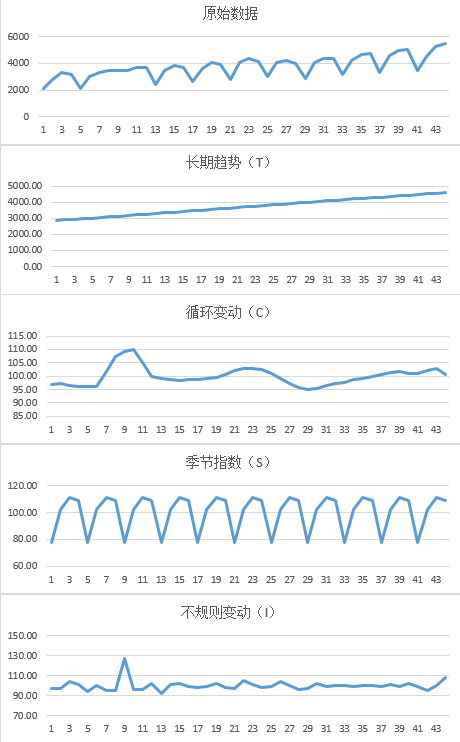应用-预测

在求得分解公式后，可以进行预测了，以第49期为例，长期趋势（T）与季节指数（S）是可以通过计算得到；循环变动可以认为在短期内不会变化为100%或者通过其他方法进行预测，比如ARIMA等；不规则变动具有随机性，因此无法进行预测，可以认为值为100%。

T49 = 2736.29 + 40.87 * 49 = 4738.92；

S1=111.15%；第49期的季节编号为1

则X49 = T49 * S1 * C * I = 4738.92 * 111.15% * 100% * 100% = 57267.31，其他期的预测如下图：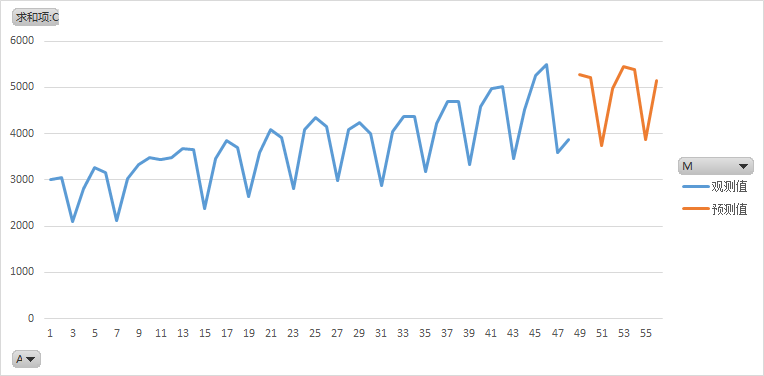应用-异常检测

此种方法也可以用于异常检测，分解后得到的不规则变动，也就是随机因素，应该是服从正太分布的，从图上也可以看出随机因素的值在100%上下波动，如果离100%相差太远，则可以认为对应的数据点有异常例如不规则变动（I）图的第9个点。


展开全文• lstm时间序列预测模型 时间序列-LSTM模型 (Time Series - LSTM Model) Advertisements 广告 Previous Page 上一页 Next Page 下一页 Now, we are familiar with statistical modelling on time series...lstm时间序列预测模型 时间序列-LSTM模型 (Time Series - LSTM Model)
Now, we are familiar with statistical modelling on time series, but machine learning is all the rage right now, so it is essential to be familiar with some machine learning models as well. We shall start with the most popular model in time series domain − Long Short-term Memory model.
现在，我们已经很熟悉时间序列的统计建模，但是机器学习现在非常流行，因此也必须熟悉某些机器学习模型。 我们将从时间序列域中最流行的模型开始-长短期记忆模型。
LSTM is a class of recurrent neural network. So before we can jump to LSTM, it is essential to understand neural networks and recurrent neural networks.
LSTM是一类递归神经网络。 因此，在进入LSTM之前，必须了解神经网络和递归神经网络。
神经网络 (Neural Networks)
An artificial neural network is a layered structure of connected neurons, inspired by biological neural networks. It is not one algorithm but combinations of various algorithms which allows us to do complex operations on data.
人工神经网络是受生物神经网络启发的连接神经元的分层结构。 它不是一种算法，而是多种算法的组合，使我们能够对数据进行复杂的操作。
递归神经网络 (Recurrent Neural Networks)
It is a class of neural networks tailored to deal with temporal data. The neurons of RNN have a cell state/memory, and input is processed according to this internal state, which is achieved with the help of loops with in the neural network. There are recurring module(s) of ‘tanh’ layers in RNNs that allow them to retain information. However, not for a long time, which is why we need LSTM models.
它是为处理时间数据而量身定制的一类神经网络。 RNN的神经元具有细胞状态/内存，并根据此内部状态处理输入，这是借助神经网络中的循环来实现的。 RNN中有“ tanh”层的重复模块，可让它们保留信息。 但是，不是很长一段时间，这就是为什么我们需要LSTM模型。
LSTM (LSTM)
It is special kind of recurrent neural network that is capable of learning long term dependencies in data. This is achieved because the recurring module of the model has a combination of four layers interacting with each other.
它是一种特殊的循环神经网络，能够学习数据的长期依赖性。 之所以能够实现这一目标，是因为模型的重复模块具有相互交互的四层组合。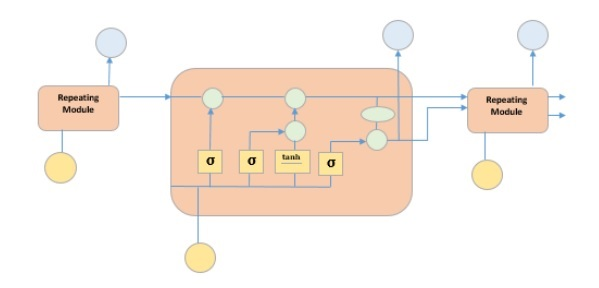The picture above depicts four neural network layers in yellow boxes, point wise operators in green circles, input in yellow circles and cell state in blue circles. An LSTM module has a cell state and three gates which provides them with the power to selectively learn, unlearn or retain information from each of the units. The cell state in LSTM helps the information to flow through the units without being altered by allowing only a few linear interactions. Each unit has an input, output and a forget gate which can add or remove the information to the cell state. The forget gate decides which information from the previous cell state should be forgotten for which it uses a sigmoid function. The input gate controls the information flow to the current cell state using a point-wise multiplication operation of ‘sigmoid’ and ‘tanh’ respectively. Finally, the output gate decides which information should be passed on to the next hidden state
上图显示了黄色方框中的四个神经网络层，绿色圆圈中的点智能算子，黄色圆圈中的输入，蓝色圆圈中的单元状态。 LSTM模块具有单元状态和三个门，这三个门为它们提供了从每个单元中选择性地学习，取消学习或保留信息的能力。 LSTM中的单元状态仅允许一些线性交互作用，从而使信息流经这些单元而不会被更改。 每个单元都有一个输入，输出和一个忘记门，可以将信息添加或删除到单元状态。 遗忘门决定使用S形函数应忘记先前单元状态中的哪些信息。 输入门分别使用“ Sigmoid”和“ tanh”的逐点乘法运算将信息流控制为当前单元状态。 最后，输出门决定应将哪些信息传递到下一个隐藏状态
Now that we have understood the internal working of LSTM model, let us implement it. To understand the implementation of LSTM, we will start with a simple example − a straight line. Let us see, if LSTM can learn the relationship of a straight line and predict it.
现在我们已经了解了LSTM模型的内部工作原理，让我们实现它。 为了理解LSTM的实现，我们将从一个简单的示例开始-一条直线。 让我们看看，LSTM是否可以学习直线的关系并对其进行预测。
First let us create the dataset depicting a straight line.
首先，让我们创建描述直线的数据集。
In :
在中：

x = numpy.arange (1,500,1)
y = 0.4 * x + 30
plt.plot(x,y)

Out:
出：

[<matplotlib.lines.Line2D at 0x1eab9d3ee10>]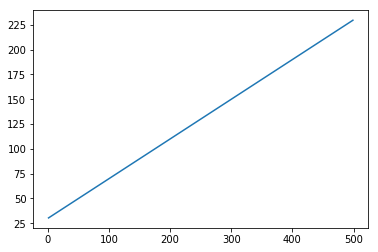In :
在中：

trainx, testx = x[0:int(0.8*(len(x)))], x[int(0.8*(len(x))):]
trainy, testy = y[0:int(0.8*(len(y)))], y[int(0.8*(len(y))):]
train = numpy.array(list(zip(trainx,trainy)))
test = numpy.array(list(zip(trainx,trainy)))

Now that the data has been created and split into train and test. Let’s convert the time series data into the form of supervised learning data according to the value of look-back period, which is essentially the number of lags which are seen to predict the value at time ‘t’.
现在已经创建了数据，并将其拆分为训练和测试。 让我们根据回溯期的值将时间序列数据转换为监督学习数据的形式，回溯期的值本质上是指可以预测时间“ t”时的滞后次数。
So a time series like this −
所以这样的时间序列-

time variable_x
t1  x1
t2  x2
:   :
:   :
T   xT

When look-back period is 1, is converted to −
当回溯期为1时，转换为-

x1   x2
x2   x3
:    :
:    :
xT-1 xT

In :
在中：

def create_dataset(n_X, look_back):
dataX, dataY = [], []
for i in range(len(n_X)-look_back):
a = n_X[i:(i+look_back), ]
dataX.append(a)
dataY.append(n_X[i + look_back, ])
return numpy.array(dataX), numpy.array(dataY)

In :
在中：

look_back = 1
trainx,trainy = create_dataset(train, look_back)
testx,testy = create_dataset(test, look_back)

trainx = numpy.reshape(trainx, (trainx.shape, 1, 2))
testx = numpy.reshape(testx, (testx.shape, 1, 2))

Now we will train our model.
现在，我们将训练模型。
Small batches of training data are shown to network, one run of when entire training data is shown to the model in batches and error is calculated is called an epoch. The epochs are to be run ‘til the time the error is reducing.
将小批量的训练数据显示给网络，一次将整个训练数据分批显示给模型并且计算出误差时的一次运行称为时期。 直到错误减少的时间段为止。
In [ ]:
在[]中：

from keras.models import Sequential
from keras.layers import LSTM, Dense

model = Sequential()
model.add(LSTM(256, return_sequences = True, input_shape = (trainx.shape, 2)))
model.compile(loss = 'mean_squared_error', optimizer = 'adam')
model.fit(trainx, trainy, epochs = 2000, batch_size = 10, verbose = 2, shuffle = False)
model.save_weights('LSTMBasic1.h5')

In :
在中：

predict = model.predict(testx)

Now let’s see what our predictions look like.
现在，让我们看看我们的预测是什么样的。
In :
在中：

plt.plot(testx.reshape(398,2)[:,0:1], testx.reshape(398,2)[:,1:2])
plt.plot(predict[:,0:1], predict[:,1:2])

Out:
出：

[<matplotlib.lines.Line2D at 0x1eac792f048>]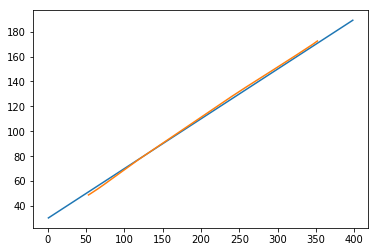Now, we should try and model a sine or cosine wave in a similar fashion. You can run the code given below and play with the model parameters to see how the results change.
现在，我们应该尝试以类似方式对正弦波或余弦波建模。 您可以运行下面给出的代码，并使用模型参数来查看结果如何变化。
In :
在中：

x = numpy.arange (1,500,1)
y = numpy.sin(x)
plt.plot(x,y)

Out:
出：

[<matplotlib.lines.Line2D at 0x1eac7a0b3c8>]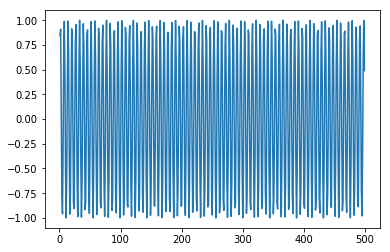In :
在中：

trainx, testx = x[0:int(0.8*(len(x)))], x[int(0.8*(len(x))):]
trainy, testy = y[0:int(0.8*(len(y)))], y[int(0.8*(len(y))):]
train = numpy.array(list(zip(trainx,trainy)))
test = numpy.array(list(zip(trainx,trainy)))

In :
在中：

look_back = 1
trainx,trainy = create_dataset(train, look_back)
testx,testy = create_dataset(test, look_back)
trainx = numpy.reshape(trainx, (trainx.shape, 1, 2))
testx = numpy.reshape(testx, (testx.shape, 1, 2))

In [ ]:
在[]中：

model = Sequential()
model.add(LSTM(512, return_sequences = True, input_shape = (trainx.shape, 2)))
model.compile(loss = 'mean_squared_error', optimizer = 'adam')
model.fit(trainx, trainy, epochs = 2000, batch_size = 10, verbose = 2, shuffle = False)
model.save_weights('LSTMBasic2.h5')

In :
在中：

predict = model.predict(testx)

In :
在中：

plt.plot(trainx.reshape(398,2)[:,0:1], trainx.reshape(398,2)[:,1:2])
plt.plot(predict[:,0:1], predict[:,1:2])

Out :
出：

[<matplotlib.lines.Line2D at 0x1eac7a1f550>]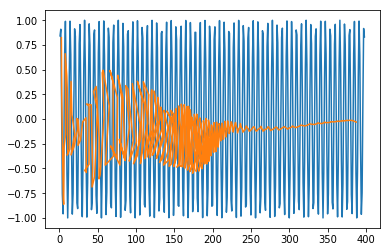Now you are ready to move on to any dataset.
现在您可以继续使用任何数据集了。
翻译自: https://www.tutorialspoint.com/time_series/time_series_lstm_model.htmlstm时间序列预测模型
展开全文神经网络 算法 python 机器学习 深度学习
• ## 算法模型---时间序列模型

万次阅读 多人点赞 2018-01-16 09:04:58
1、时间序列时间序列时间间隔不变的情况下收集的不同时间点数据集合，这些集合被分析用来了解长期发展趋势及为了预测未来。...常用的时间序列模型有AR模型、MA模型、ARMA模型和ARIMA模型等。2、时间
文章来源
时间序列
时间序列是时间间隔不变的情况下收集的不同时间点数据集合，这些集合被分析用来了解长期发展趋势及为了预测未来。
时间序列与常见的回归问题的不同点在于:
1、时间序列是跟时间有关的;而线性回归模型的假设：观察结果是独立的在这种情况下是不成立的。
2、随着上升或者下降的趋势，更多的时间序列出现季节性趋势的形式；
常用的时间序列模型有AR模型(Autoregressive model:自回归模型)、MA模型(moving average model:滑动平均模型)、ARMA模型(Auto-Regressive and Moving Average Model:自回归滑动平均模型)和ARIMA模型(Autoregressive Integrated Moving Average Model:自回归积分滑动平均模型)等。
时间序列的预处理(使数据平稳化和随机化)
拿到一个观察值序列之后，首先要对它的平稳性和纯随机性进行检验，这两个重要的检验称为序列的预处理。根据检验的结果可以将序列分为不同的类型，对不同类型的序列我们会采用不同的分析方法。
平稳化处理
平稳 就是围绕着一个常数上下波动且波动范围有限，即有常数均值和常数方差。如果有明显的趋势或周期性，那它通常不是平稳序列。序列平稳不平稳，一般采用三种方法检验：
时序图检验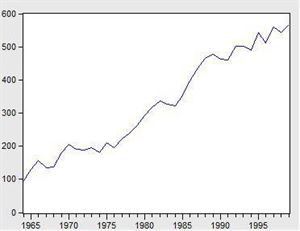看看上面这个图，很明显的增长趋势，不平稳。
利用自相关系数和偏相关系数
自相关系数和偏相关系数的概念可参考《算法模型— 概率论基础—相关系数相关》
下面是不平稳数据的自相关和偏相关系数的一种情形。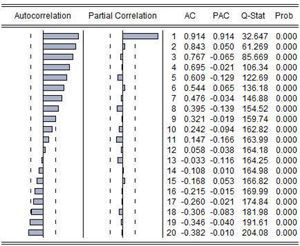左边第一个为自相关图（Autocorrelation），第二个偏相关图(Partial Correlation)。
平稳的序列的自相关图和偏相关图要么拖尾，要么是截尾。截尾就是在某阶之后，系数都为 0 。怎么理解呢，看上面偏相关的图，当阶数为 1 的时候，系数值还是很大， 0.914;二阶长的时候突然就变成了 0.050. 后面的值都很小，认为是趋于 0 ，这种状况就是截尾。什么是拖尾，拖尾就是有一个缓慢衰减的趋势，但是不都为 0 。
自相关图既不是拖尾也不是截尾。以上的图的自相关是一个三角对称的形式，这种趋势是单调趋势的典型图形，说明这个序列不是平稳序列。
平稳序列的自相关系数会快速衰减。
单位根检验
单位根检验是指检验序列中是否存在单位根，如果存在单位根就是非平稳时间序列。
利用差分将序列数据转换为平衡序列
差分可以将数据转换为平稳序列。
一阶差分指原序列值相距一期的两个序列值之间的减法运算；k阶差分就是相距k期的两个序列值之间相减。如果一个时间序列经过差分运算后具有平稳性，则该序列为差分平稳序列，可以使用ARIMA模型进行分析。
确定不平稳后，依次进行1阶、2阶、3阶…差分，直到平稳为止。
随机化处理
对于纯随机序列，又称白噪声序列，序列的各项数值之间没有任何相关关系，序列在进行完全无序的随机波动，可以终止对该序列的分析。白噪声序列是没有信息可提取的平稳序列。对于平稳非白噪声序列，它的均值和方差是常数。通常是建立一个线性模型来拟合该序的发展，借此提取该序列的有用信息。ARMA模型是最常用的平稳序列拟合模型。
平稳时间序列建模
某个时间序列经过预处理，被判定为平稳非白噪声序列，就可以进行时间序列建模。
建模步骤：
（1）计算出该序列的自相关系数（ACF）和偏相关系数（PACF）；
（2）模型识别，也称模型定阶。根据系数情况从AR§模型、MA(q)模型、ARMA(p，q)模型、ARIMA（p，d，q）模型中选择合适模型，其中p为自回归项，d为差分阶数，q为移动平均项数。
若平稳序列的偏相关函数是截尾的，而自相关函数是拖尾的，可断定序列适合AR模型；若平稳序列的偏相关函数是拖尾的，而自相关函数是截尾的，则可断定序列适合MA模型；若平稳序列的偏相关函数和自相关函数均是拖尾的，则序列适合ARMA模型。（截尾是指时间序列的自相关函数（ACF）或偏自相关函数（PACF）在某阶后均为0的性质（比如AR的PACF）；拖尾是ACF或PACF并不在某阶后均为0的性质（比如AR的ACF）。）
（3）估计模型中的未知参数的值并对参数进行检验；
（4）模型检验；
（5）模型优化；
（6）模型应用：进行短期预测。
混合自回归移动平均过程（公式表达）
差分方程
所谓差分方程即将变量 $y_t$与它的滞后期联系起来的表达式。
研究变量在第t期的值记为$y_t$。假定给出的动态方程将变量y第t期的值与另外的变量$w_t$以及y的前一期联系起来:
$y_t=ϕy_{t−1}+w_t$
上述称为一阶差分方程是因为仅仅只有变量的一阶滞后$(y_t−1)$出现在方程中。
移动平均
“移动平均”的含义源于$Y_t$是最近两期的$\epsilon$的加权平均。
令${\epsilon_t}$是一个白噪声序列。
$Y_t=μ+\epsilon_t+θ\epsilon_{t−1}$
其中μ和θ可以是任意的常数。这个时间序列称为一阶移动平均过程，记为$MA(1)$。
自回归过程
一阶自回归过程
一个一阶自回归，记作$AR(1)$满足下面的差分方程
$Y_t=c+ϕY_{t−1}+\epsilon_t$
${\epsilon_t}$是一个白噪声序列。
p阶自回归过程
一个p阶自回归，记作$AR(p)$满足下式
$Y_t=c+ϕ_1Y_{t−1}+ϕ_2Y_{t−2}+...+ϕ_pY{t−p}+\epsilon_t$
混合自回归移动平均过程
一个$ARMA(p,q)$过程包括自回归和移动平均项：
$Y_t=c+ϕ_1Y_{t−1}+ϕ_2Y_{t−2}+...+ϕ_pY{t−p}+\epsilon_t+θ_1ϵ_{t−1}+θ_2ϵ{t−2}+...+θ_qϵ_{t−q}$
利用statsmodels库实现时间序列的分析处理
Time Series analysis tsa
statsmodels.tsa.arima_model.ARIMA
AR(I)MA时间序列建模过程——步骤和python代码
Seasonal ARIMA with Python
Python 3中使用ARIMA进行时间序列预测的指南
Python_Statsmodels包_时间序列分析_ARIMA模型
时间序列实战（一）
How to Make Predictions for Time Series Forecasting with Python
How to Use and Remove Trend Information from Time Series Data in Python
时间序列分析之ARIMA上手-Python
[python] 时间序列分析之ARIMA
python中有专门的库statsmodels可以用来作时间序列分析，但spark中则没有现成的。
#-*- coding: utf-8 -*-
import matplotlib.pyplot as plt
import pandas as pd
b=data['fData']
plt.plot(data['fData'])
plt.show()

数据结构如下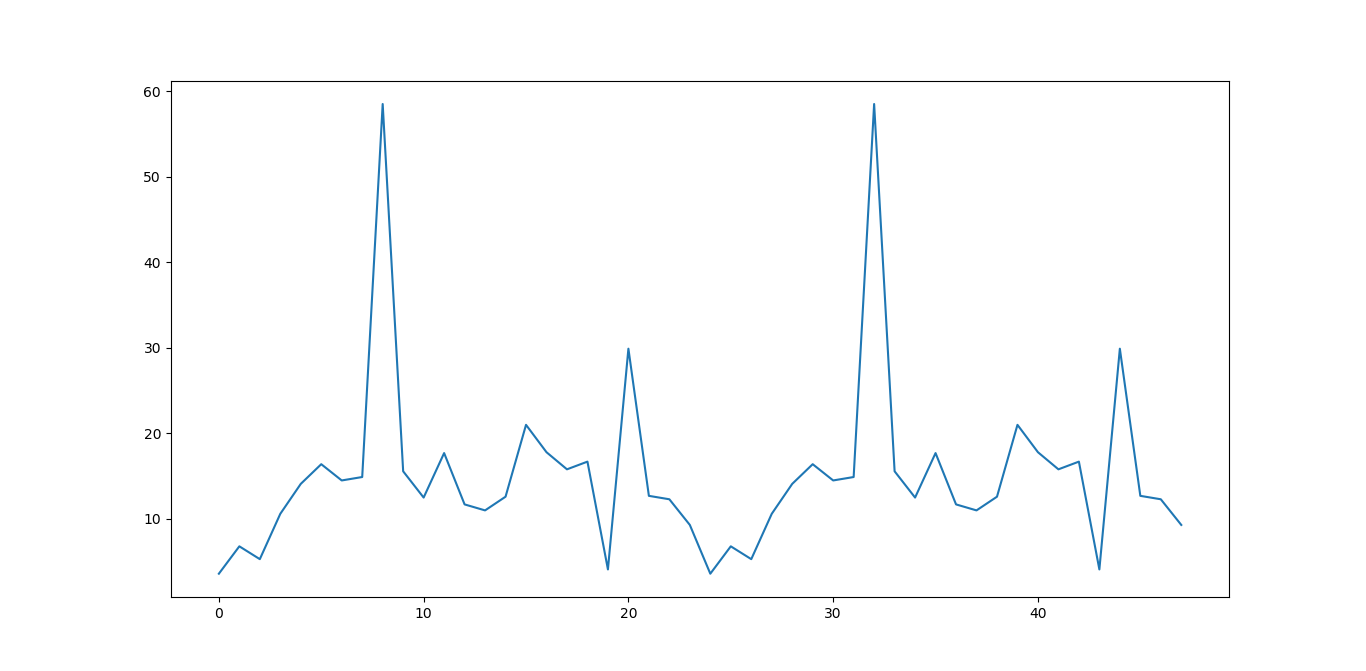#自相关图
from statsmodels.graphics.tsaplots import plot_acf
plot_acf(data['fData']).show()

如下所示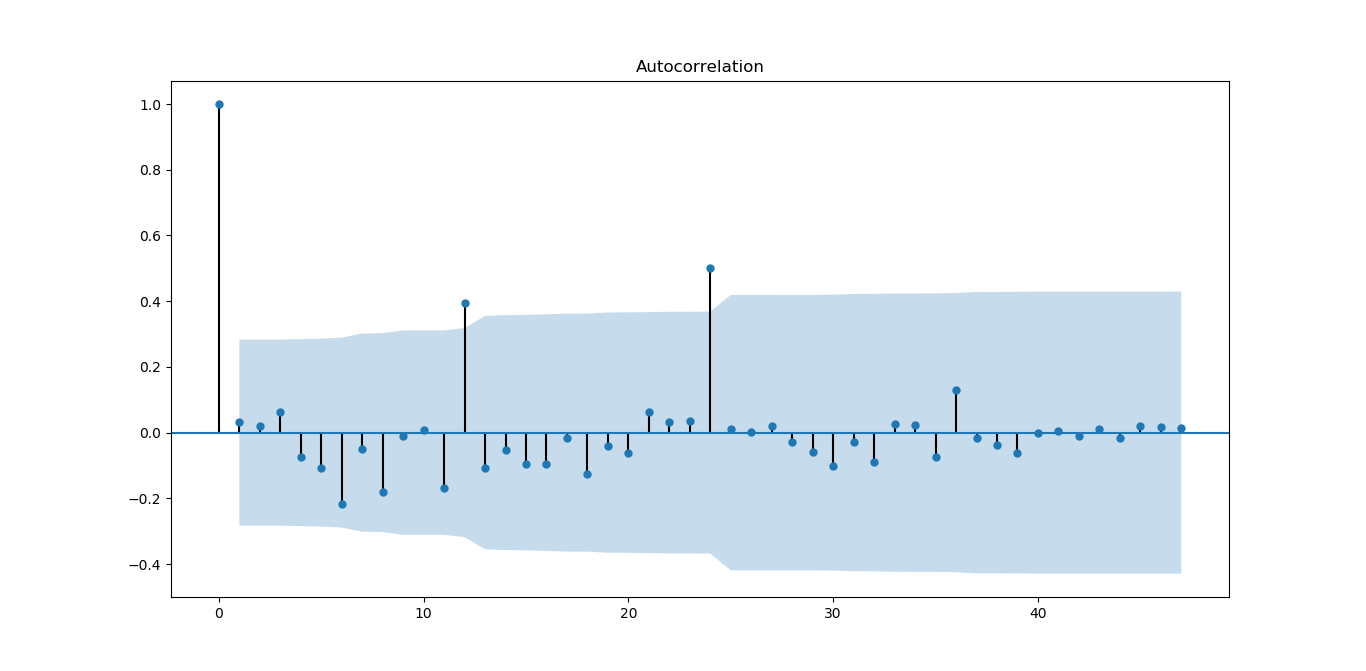从图上可以自相关系数基本是拖尾的，稍做调整就可以使用。
#偏自相关图
from statsmodels.graphics.tsaplots import plot_pacf
plot_pacf(data['fData']).show()

如下图所示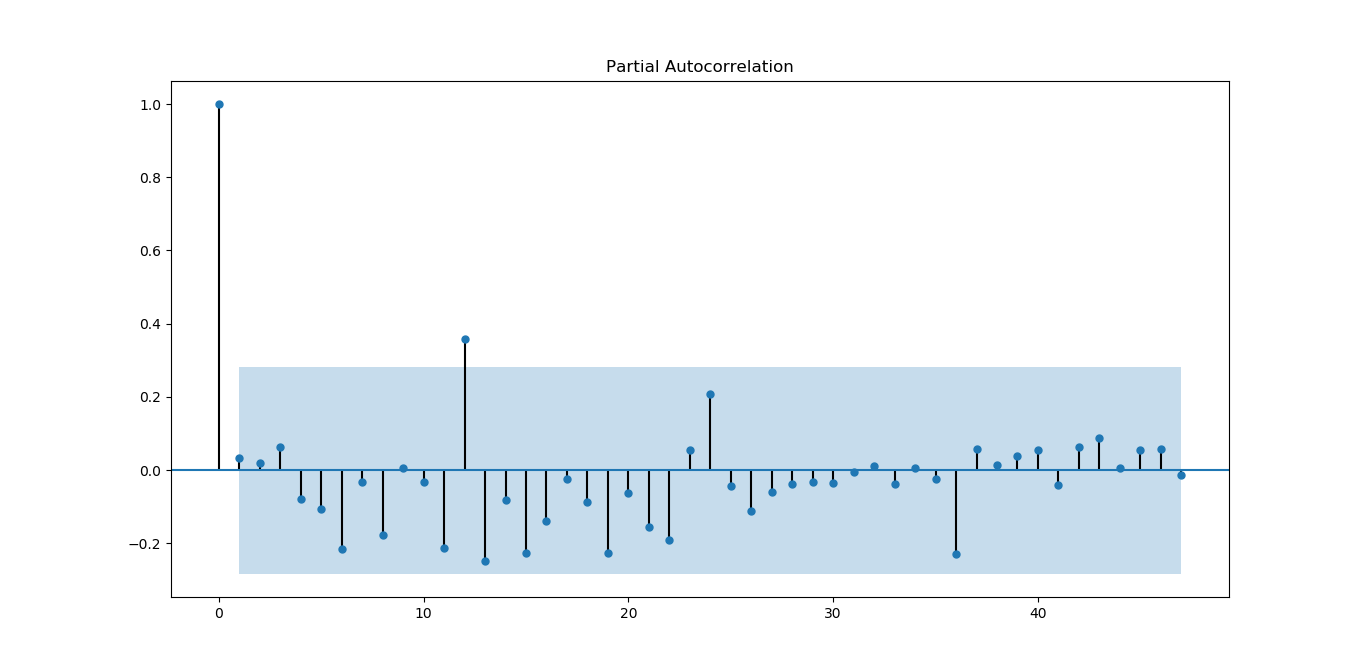偏相关系数也基本符合拖尾。
#平稳性检测

如下所示
原始序列的ADF检验结果为：
(-6.561077625309946, 8.378411469638636e-09, 0L, 47L, {'5%': -2.925338105429433, '1%': -3.5778480370438146, '10%': -2.6007735310095064}, 273.5266547319719)

p值远小于0.05，拒绝原假设（原假设认为存在单位根），所以该序列为平衡时间序列。
#白噪声检验
from statsmodels.stats.diagnostic import acorr_ljungbox
print('差分序列的白噪声检验结果为：')
print(acorr_ljungbox(data['fValueData'], lags=1))

结果如下
差分序列的白噪声检验结果为：
(array([0.05198092]), array([0.81965149]))

P=0.81965149，统计量的P值大于显著性水平0.05，则接受原假设（原假设认为为白噪声序列）。
下面对原序列做一阶差分运算
#差分后的结果
D_data = data['fValueData'].diff().dropna()
D_data.columns = ['fValueData']
#时序图
D_data.plot()

结果如下：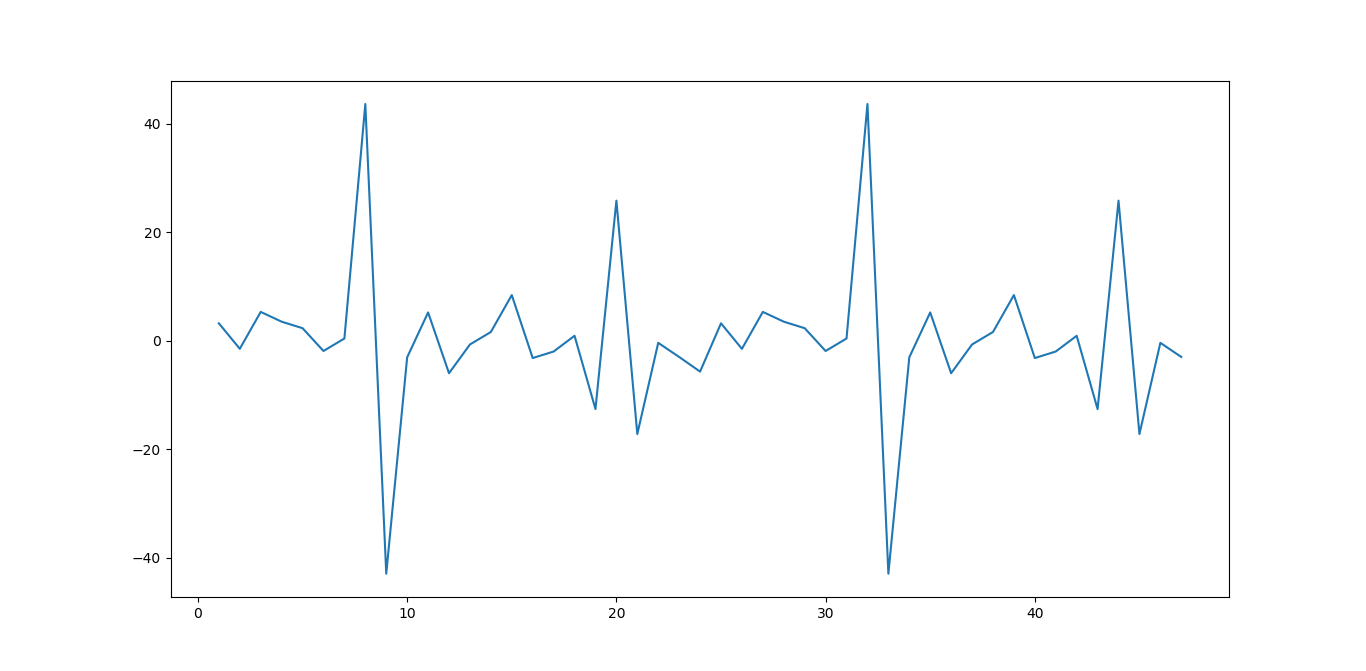从图上看，一阶差分后，感觉数据分布更对称为随机了。
#自相关图
plot_acf(D_data).show()
plt.show()

结果如下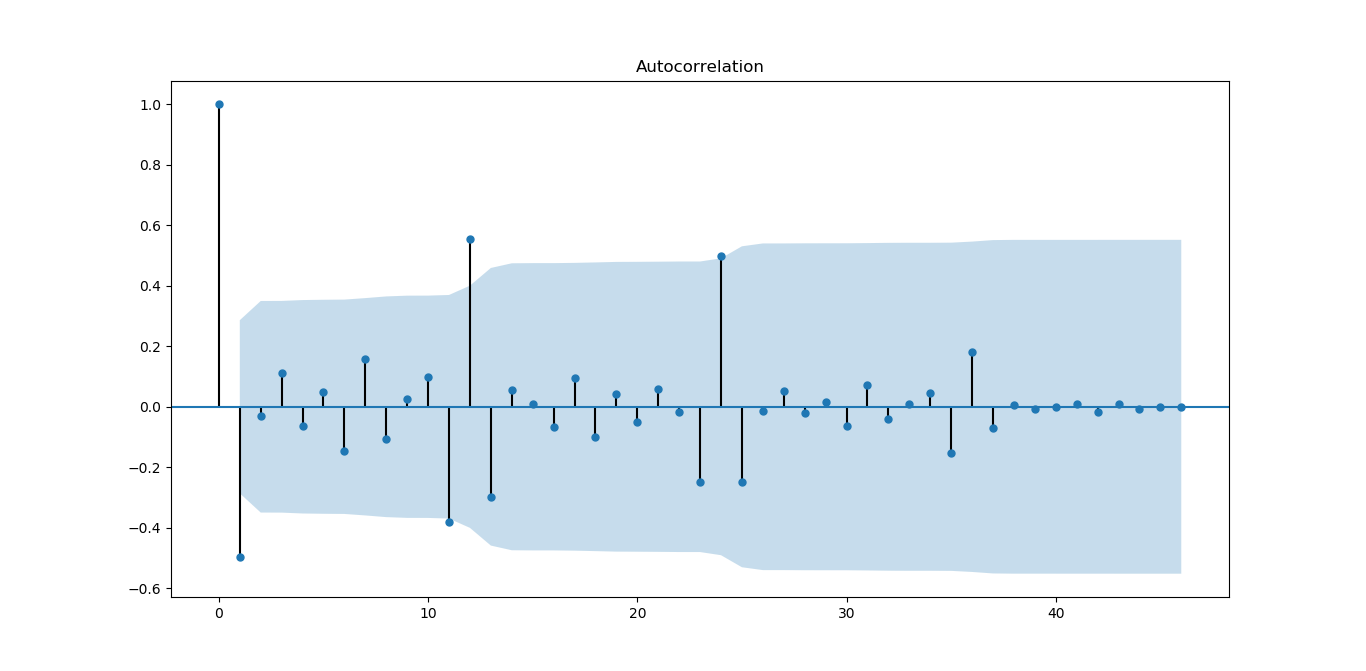从图上看，一阶差分对数据有改善，振荡衰减更明显
#偏自相关图
plot_pacf(D_data).show()

结果如下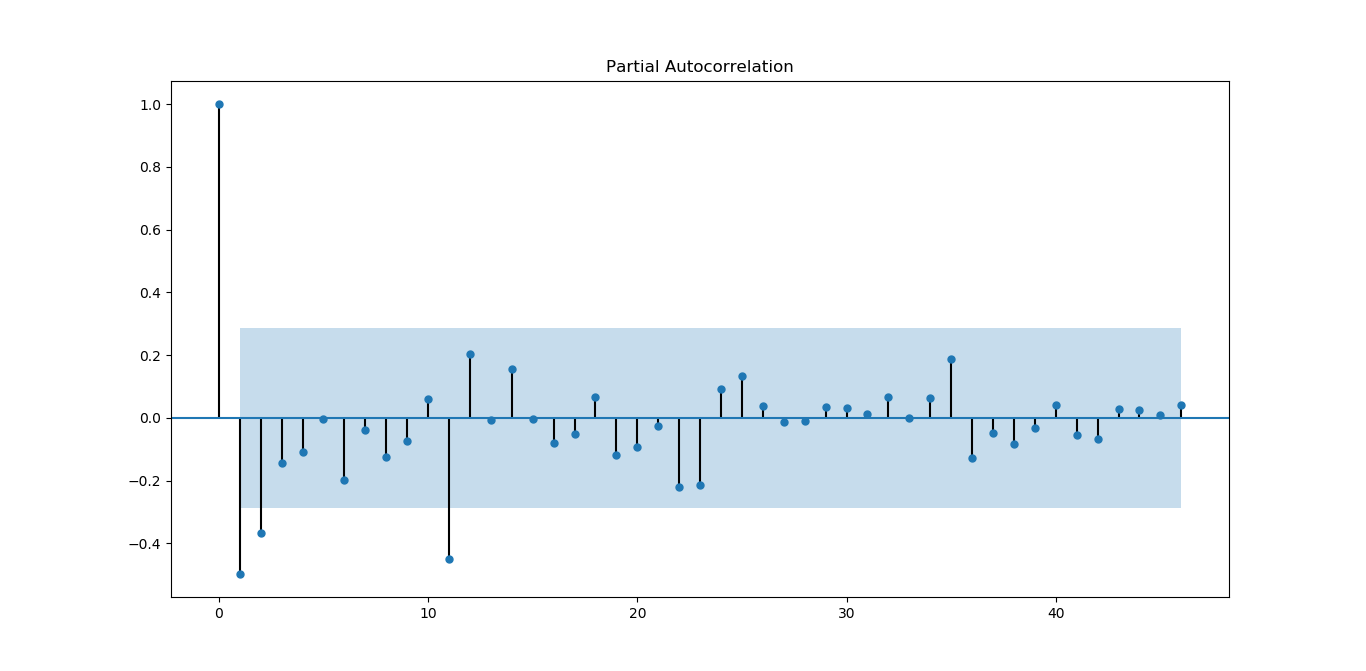从图上看，偏相关系数虽然没有自相关系数好，但基本能满足平稳序列的要求。
#平稳性检测

结果如下
差分序列的ADF检验结果为：
(-4.595500765524432, 0.0001316587309452837, 10L, 36L, {'5%': -2.9459512825788754, '1%': -3.626651907578875, '10%': -2.6116707716049383}, 270.35268975914374)

p值远小于0.05，拒绝原假设（原假设认为存在单位根），所以该序列为平衡时间序列。
#白噪声检验
from statsmodels.stats.diagnostic import acorr_ljungbox
#返回统计量和p值
print('差分序列的白噪声检验结果为：')
print(acorr_ljungbox(D_data, lags=1))

结果如下：
差分序列的白噪声检验结果为：
(array([12.35619393]), array([0.00043953]))

P=0.00043953，统计量的P值小于显著性水平0.05，则拒绝原假设（原假设认为为白噪声序列），所以一阶差分后该序列为非白噪声序列，有进一步分析的价值。
Holt-Winters模型原理及python实现
本部分来源，也可以参考该文
原理分析
公式前面也写过，这里再表述一下，有时间再来整理
假设随机时间序列为：
$x_t(t=1,2,\ldots,N,N=n)$
时间序列数据一般有以下几种特点：1.趋势(Trend)  2. 季节性(Seasonality)。
趋势描述的是时间序列的整体走势，比如总体上升或者总体下降。下图所示的时间序列是总体上升的：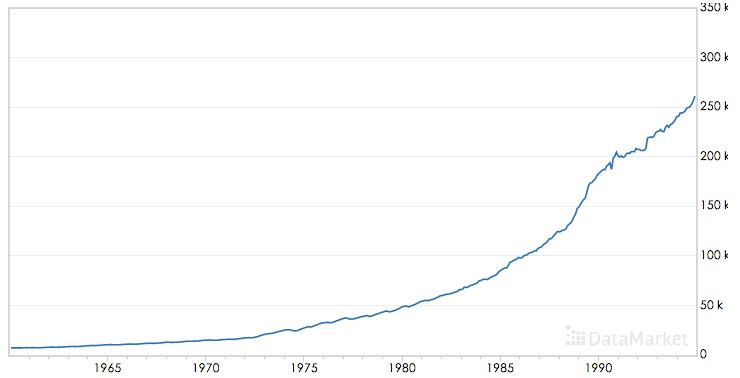季节性描述的是数据的周期性波动，比如以年或者周为周期，如下图：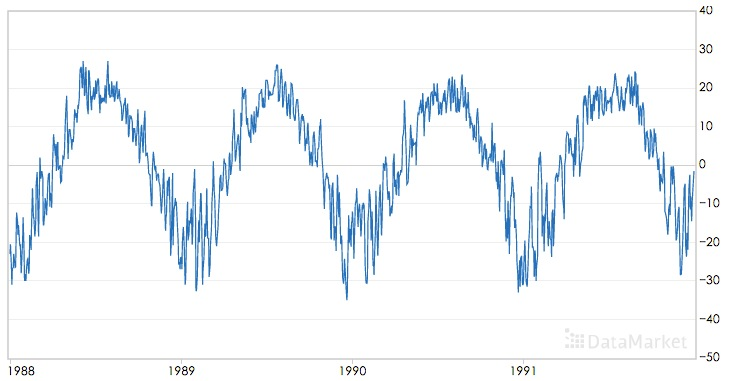三次指数平滑算法可以对同时含有趋势和季节性的时间序列进行预测，该算法是基于一次指数平滑和二次指数平滑算法的。
移动平均(The simple moving average (MA))
直观上,最简单的平滑时间序列的方法是实现一个无权重的移动平均,目前已知的方法是用窗口函数,平滑统计量 $S_t$就是最近k个观察值的均值。公式如下:
$s_t=\frac{1}{k} \sum_{n=0}^{k-1} x_{t-n}=\frac{x_t+x_{t-1}+\ldots + x_{t-k+1}}{k}=s_{t-1}+\frac{x_t -x_{t-k}}{k}$
这样的方法存在明显的缺陷,当k比较小时,预测的数据平滑效果不明显,而且突出反映了数据最近的变化;当k较大时,虽然有较好的平滑效果,但是预测的数据存在延迟。而且最少需要k个值(窗口有限)。
加权移动平均
一种稍微复杂的方法是先选择一组权重因子来计算加权移动平均
${\omega_1,\omega_2,\ldots,\omega_k},满足\sum_{n=1}^{k}\omega_n=1$
然后用这些权重来计算这些平滑统计量:
$s_t=\sum_{n=1}^{k}\omega_n x_{t-n+1}=\omega_1x_t+\omega_2 x_{t-1}+\ldots+\omega_{k}x_{t-k+1}$
在实践中,通常在选择权重因子时,赋予时间序列中的最新数据更大的权重,并减少对旧数据的权重。这个方法也需要最少k个值,并且计算复杂。
简单指数平滑法
幸运地是有一种方法可以避免上述问题,它叫做指数平滑法。最简单的指数平滑法如下:
$s_i = \alpha x_i + (1-\alpha)s_{i-1}$
其中$\alpha$是平滑因子,$0 < \alpha < 1$。$s_i$是当前时刻的平滑值。换句话说,平滑统计值$S_i$是当前统计值$X_t$与上一时间平滑值$S_{i-1}$加权平均。这个简单指数平滑是很容易被应用的,因为只要有两个观察值就能计算了。这里α的选取,我们可以采用最小二乘来决定$\alpha$(最小化$(s_t-x_t)^2$)。
一次指数平滑算法进行预测的公式为：
$x_{i+h}=s_i$
其中i为当前最后的一个数据记录的坐标，亦即预测的时间序列为一条直线，不能反映时间序列的趋势和季节性。
为什么被称为“指数”平滑法
从它的递推公式就能发现:
简单指数平滑法适用于没有总体趋势的时间序列。如果用来处理有总体趋势的序列,平滑值将往往滞后于原始数据,除非α的值接近1,但这样一来就会造成不够平滑。
二次指数平滑（HoltWinters-无季节趋势）
为了解决上述问题,于是引出了二次指数平滑,能够保留总体趋势信息。因为将指数平滑应用了两次,所以被称为二次指数平滑。与简单指数平滑相比,二次指数平滑加入了时间趋势统计量$t_i$,公式如下:
$s_i=\alpha x_i + (1-\alpha)(s_{i-1}+t_{i-1})\\ t_i=\beta (s_i-s_{i-1}) + (1-\beta)t_{i-1}$
$s_i$添加了与一次指数平滑相比，添加了一个新的趋势项$t_i$；而$t_i$的表达式与一次指数平滑形式上是一样的，只不过用将$x_i$换成$(s_i-s_{i-1})$,趋势可以想像成增量，就是在此刻与上一刻的差值，趋势当然应该用平滑后的数据的差值；同时将一次平滑中的$s_{i-1}$换成$t_{i-1}$
二次指数平滑的预测公式为
$x_{i+h}=s_i+h \ t_i$
二次指数平滑的预测结果是一条斜的直线
三次指数平滑
三次指数平滑将时间序列的季节性这一特征也考虑进去了。
如果每个12月都比每个11月多卖出1000套公寓,我们就说这样的季节趋势是“累加性”的。可以用绝对增⻓来表示。如果我们在夏季比冬季多卖出10%的公寓,那么季节趋势在自然中是“累乘性”的。
累乘性公式如下:
下面的式子中$k$表示季节频率，这个还需要查资料进行理解
$s_i=\alpha \frac{x_i }{p_{i-k}} +(1-\alpha)(s_{i-1}+t_{i-1})\\ t_i=\beta (s_i-s_{i-1}) + (1-\beta)t_{i-1}\\ p_i=\gamma \frac{x_i}{s_i} + (1-\gamma)p_{t-k}\\$
上式中k为周期， 累乘三次指数平滑的预测公式为：
$x_{i+h}=(s_i+h\ t_i)p_i-k+(h\ mod\ k)$
或者
$x_{i+h}=(s_i+h\ t_i)p_{i-k+1+(h-1) \ mod \ k}$
两个公式还需要确认下
累乘性公式初始值的计算
$s_k=\frac{1}{k} (s_1+s_2+\cdots+s_k)\\ t_k=\frac{1}{k}\left[ \frac{x_{k+1}-x_1}{k}+\frac{x_{k+2}-x_2}{k}+\cdots+\frac{x_{k+k}-x_k}{k} \right]\\ p_1=\frac{x_1}{s_k},p_2=\frac{x_2}{s_k},\cdots,p_k=\frac{x_k}{s_k}$
累加性公式如下:
$s_i=\alpha (x_i-p_{i-k} )+(1-\alpha)(s_{i-1}+t_{i-1})\\ t_i=\beta (s_i-s_{i-1}) + (1-\beta)t_{i-1}\\ p_i=\gamma (x_i-s_i) + (1-\gamma)p_{i-k}\\ x_{i+h}=s_i+hb_i + p_{i-k+h \ mod \ k}$
或者
$x_{i+h}=s_i+hb_i + p_{i-k+1+(h-1) \ mod \ k}$
两个公式还需要确认下
累加性公式初始值的计算
$s_k=\frac{1}{k} (s_1+s_2+\cdots+s_k)\\ t_k=\frac{1}{k}\left[ \frac{x_{k+1}-x_1}{k}+\frac{x_{k+2}-x_2}{k}+\cdots+\frac{x_{k+k}-x_k}{k} \right]\\ p_1=x_1-s_k,p_2=x_2-s_k,\cdots,p_k=x_k-s_k$
其中 $\alpha$是数据平滑因子, $0 < \alpha < 1$;$\beta$是趋势平滑因子,$0 < \beta < 1$; $\gamma$是季节改变平滑因子$0 < \gamma < 1$。
$\alpha，\beta，\gamma$的值都位于[0,1]之间，可以多试验几次以达到最佳效果。
$s,t,p$初始值的选取对于算法整体的影响不是特别大，通常的取值为$s_0=x_0,t_0=x_1-x_0$,累加时$p=0$,累乘时$p=1$.
对三次指数平滑法而言,我们必须初始化一个完整的“季节”$p_i$的值,不过我们可以简单地设置为全1(针对累乘式)或全0(针对累加式)。只有当序列的⻓度较短时,我们才需要慎重考虑初始值的选取。
我们这里讲的Holt-Winters模型就是三次指数平滑法。哇,终于切入正题了。
所有的指数平滑法都要更新上一时间步⻓的计算结果,并使用当前时间步⻓的数据中包含的新信息。它们通过“混合”新信息和旧信息来实现,而相关的新旧信息的权重由一个可调整的拌和参数来控制。各种方法的不同之处在于它们跟踪的量的个数和对应的拌和参数的个数。三次指数平滑法,功能最强大,既能体现趋势性又能体现季节性,所以三次指数平滑法的参数最多,有三个。
下图为使用累加三次指数平滑进行预测的效果：其中红色为源时间序列，蓝色为预测的时间序列，$\alpha，\beta，\gamma$的取值为0.45，0.2，0.95：下图为累乘三次指数平滑进行预测的效果，$\alpha，\beta，\gamma$的取值为0.4，0.05，0.9：可以看到三次指数平滑算法可以很好的保存时间序列数据的趋势和季节性信息，在International Airline Passengers数据集上累乘平滑指数算法的效果更好。
python代码实现
我们知道HoltWinters模型有三个可调参数,我们的目的就是训练出有效的α,β, γ
。我们有两种方法,一种就是自己取值来试试,一种就是采用数值优化的思想,比如
前面我们提到的最小二乘来最小化误差来求参数(注意不一定能全局收敛!这个问题
实在是让人头痛。。。)我们就采用最小二乘法(L-BFGS)。
RMSE的实现
statsmodels中的holtwinters
statsmodels.tsa模块
statsmodels.tsa.holtwinters源码
statsmodels.tsa.holtwinters.ExponentialSmoothing
ExponentialSmoothing - 指数平滑算法
基于SVM作短期时间序列的预测
传统的做法是提取1、2、3、4、5、7、9、13个单位时间的数据作为特征进行预测；
举个例子进行分析，比如每天都有口香糖的销量，那么如何通过几周的数据预测明天的数据，
就可以选择前1、2、3、4、5、7、14天的数据作为特征，从而预测明天的数据，
通过构建特征，再选择核函数进行预测，其中调参的参数尽量要进行最优化，
参考方法：如果选择RBF核函数，那么其中就会有三个参数，固定两个，然后不停的优化另外一个，直到得到最优解。
具体应用的例子：
（2）SVM预测股票指数：基于SVM修正的模糊时间序列模型在沪指预测中的应用
（3）SVM预测时间序列其他方面：http://www.docin.com/p-233353900.html
LSTM模型分析及对时序数据预测的具体实现（python实现）
来源：2017年09月30日 10:28:08
如何用LSTM自编码器进行极端事件预测？(含Python代码)
时间序列的并行实现
spark里面的库是没有时间序列算法的，但是国外有人已经写好了相应的算法。其github网址是:https://github.com/sryza/spark-timeseries
sryza/spark-timeseries
Spark-TimeSeries使用方法
A New Library for Analyzing Time-Series Data with Apache Spark
【Spark Summit East 2017】使用Spark进行时间序列分析
基于小波变换的时间序列预测，Python实现，来自雪球
清华AIOps算法：KPI聚类
其他资料
时间序列挖掘-预测算法-三次指数平滑法(Holt-Winters)
Holt-Winters原理和初始值的确定
时间序列模型Prophet使用详细讲解


展开全文• ## 时间序列模型

千次阅读 2018-12-04 20:40:42
1. 时间序列分析方法概述  一个时间序列往往是一下几类变化形式的叠加或耦合。  （1）长期趋势变动：反映主要变化趋势； ...常见的时间序列模型有：  （1）加法模型： Yt = Tt + St + Ct...
• 时间序列预测模型 1.时间序列分解 2.ARIMA模型 时间序列（或称动态数列）是指将同一统计指标的数值按其发生的时间先后顺序排列而成的数列。时间序列分析的主要目的是根据已有的历史数据对未来进行预测。 一个时间...数据分析
• 实验 名称 疏系数模型 和季节模型 ... SPSS建立时间序列乘法季节模型实战案例 Python建立时间序列ARIMA模型实战案例 疏系数模型的定义 时序图 白噪声检验 ...机器学习机器学习
• 数据来自澳大利亚莫纳什大学统计学教授Rob Hyndman创建的时间序列数据库（TSDB），数据库涵盖近800个时间序列数据（经济、水文、气象、农业等）； 网址：http://datamarket.com/data/list/?q=provider:tsdl 数据集...
• 今天学习了一下时间序列模型中的指数平滑模型。 时间序列数据的常用模型包括：Holt-Winters Model以及著名的Arima Model。 时间序列模型一般包含3种components: Trend Component Seasonal Component Irregular ...R
• 实验 名称 疏系数模型 和季节模型 实验 ... 1、简单季节模型 ... 1、掌握疏系数模型 ... 2、熟练建立季节模型 ...简单季节模型结构 ... SPSS建立时间序列乘法季节模型实战案例 Pyth...
• ## 时间序列模型Prophet使用详细讲解

万次阅读 多人点赞 2018-10-26 20:39:34
之前我们已经讲过了如何Windows系统下安装Python版本的...教程使用的数据集可 Prophet 的 github 主页 的 examples 文件夹 内下载得到。 目录 一、简易入门 二、饱和预测 2.1 预测饱和增长 2....Prophet
• 简介 时间序列预测法是一种历史资料延伸预测，也称历史引伸预测法...时间序列预测法就是通过编制和分析时间序列，根据时间序列所反映出来的发展过程、方向和趋势，进行类推或延伸，借以预测下一段时间或以后若干年内可机器学习
• 时间序列分析的AR模型是利用历史数据来预测未来数据的一种模型，也叫做自回归模型auto-regressive。滤波器也有应用，原理跟FIR滤波一样，如果是用matlab的话使用相同AR系数预测的序列和FIR滤波器函数得到的...最小二乘法估计 FIR滤波器
• 1 时间序列预测模型 1.1 时间序列分解 时间序列是指将同一 统计量的数值按其发生的时间先后顺序排列而成的数列，常用按时间顺序排列的一组随机变量????1,????2,⋯????????,⋯表示 一个随机事件的时间序列，简记为 {?...
• 阻尼趋势模型模型五：简单季节性模型模型六：温特加法模型模型七：温特乘法模型三、一元时间序列分析的模型1、基本的理解2、一元时间序列分析的模型：AR( P)模型3、一元时间序列分析的模型：MA(Q)模型4、ARMA(p.q)...
• 时间序列的分析的步骤是先对数据进行平稳性和非白噪声检验（如不满足需对数据进行平滑或差分等预处理），然后才是模型调参跟预测，因此本文分为2大部分介绍，答题思路见脑图。 一、数据准备&探索 1、平稳性 ...python
• 第1部分_时间序列分析的概念与时间序列分解模型 时间序列 时间序列的基本概念 区分时期和时点序列 时期序列适用于灰色预测模型 时间序列分解 长期变动趋势 T 季节趋势 S 第2部分_SPSS七种指数平滑方法的简单...
• **《时间序列分析》**是统计学的一个非常重要的分支，是以概率论与数理统计为基础、计算机应用为技术支撑，迅速发展起来的一种应用性很强的科学方法。时间序列是变量按时间间隔的顺序而下形成的随机变量序列，大量...python 数据分析
• 目录一、方法简介数据示例二、ARIMA模型python建模过程[^2]1 添加基础库2 读取数据3 绘制时间序列图4 自相关5 平稳性检验6 时间序列的差分d7 合适的p,q8 模型检验Ljung-Box检验9 模型预测 时间序列分析方法1主要有...
• 使用时间序列分解模型预测商品销量1.1实验目的1.2实验概述1.3 实验目标1.4 实验工具1.5 实验准备实验资源============== 这是一条分割线 ==============必看：想要直接开始操作点击这里 发布本博客皆是为了做笔记，...
• 充分考虑未来各种影响因素的基础上，根据历史销量以及市场上对产品需求的变化情况，对未来一定时期内产品的产品销量变化所进行的科学预计和推测。 二、商品销量预测三大特点： 1.连贯性:把过去、现在与未来的发展...
• 一、价格弹性时间序列分解模型预测商品销量 1. 课程目标 (1) 掌握商品销量预测的相关概念 (2) 了解预测方法的适用场景 (3) 阿里云产品构建商品销量预测模型 (4) 根据预测模型制定商品运营策略 (5)目录 - 商品销售...
• 1 时间序列 时间序列是指同一种现象不同时间上...估计时间序列模型之前需把不平稳的时间序列转化为平稳序列。判断一个时间序列的平稳性可通过数据图和自相关函数图，如果数据图呈现线性或二次趋势形状，则该时间R语言 卡尔曼滤波器
• 无意发现了一个巨牛的人工智能教程，忍不住分享一下给大家。教程不仅是零基础，通俗易懂，...在回归里面，我们有一元回归于多元回归，在时间序列里面，我们有自回归。与一元、多元一样，我们分为一阶与多阶自回...R
• 文章目录前言一、确定性时间序列分析二、使用步骤1.引入库2.读入数据总结 前言 时间序列是按时间顺序排列的、随时间变化且相互关联的数据序列。分析时间序列的方法构成数据分析的一个重要领域，即时间序列分析。 1......